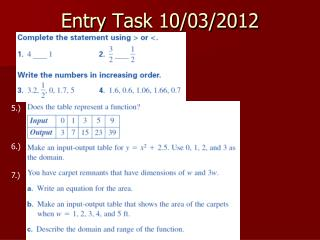DownloadDownload PresentationDownload Presentation- - - - - - - - - - - - - - - - - - - - - - - - - - - E N D - - - - - - - - - - - - - - - - - - - - - - - - - - -
##### Presentation Transcript

1. Entry Task 10/03/2012 5.) 6.) 7.)

2. Algebra 1Section 2.1 Objective: Graph and compare real numbers using a number line.

3. Vocabulary • Real Number- all numbers except imaginary numbers • Real Number Line- a horizontal line used to picture real numbers • Origin- the point labeled zero on the number line • Integers- whole numbers plus the opposite of each whole number and zero • The opposite of a number is the number that is the same distance from zero on the other side of the number line. • Ex. The opposite of 2.5 is -2.5 • The absolute value of a number is the distance that number is from zero on the number line. Absolute value of x is notated • the absolute value of -2.3 is 2.3 and the absolute value of 4 is 4.

4. Graphing Real Numbers • Graph the numbers 2 and -4 on the number line

5. Opposite of a Number • The opposite of a number is the number that is the same distance from zero on the other side of the number line. • Ex. Find the opposite of 2.5 The opposite of 2.5 is -2.5

6. Absolute Value • The absolute value of a number is the distance that number is from zero on the number line. Absolute value of x is notated • Find the absolute value of -2.3 and 4. The absolute value of -2.3 is 2.3 and the absolute value of 4 is 4.

7. Entry Task 10/04/2011 • Get out your notebook. • You may use: • A calculator • Your notebook • Your knowledge folder • When you are done work on finishing 1.7 and 2.1

9. Entry Task 10/06/2011 • 1.) write the statement as an expression “3 more than the product of 4 and a number n.” • write the sentence as an equation or inequality 2.) “Fourteen plus the product of twelve and a number y is less than or equal to fifty.” 3.) A number x squared plus forty-four is equal to the number x to the fourth power times three. • Describe the domain and range of the function y=2x-4

10. Algebra 1Section 2.2 and 2.3 Objectives: Add real numbers and subtract real numbers.

11. Properties of Additionwrite these down and then come up with an example for each • Commutative Property- The order in which two numbers are added does not change the sum. i.e. a+b = b+a • Associative Property- The way you group addition does not change the sum. i.e. (a+b)+c = a+(b+c) • Identity Property- The sum of a number and 0 is the number. i.e. a+0 = a • Inverse Property- the sum of a number and the opposite of the number is 0. i.e. a+(-a) = 0

12. Subtraction Rule • To subtract b from a, add the opposite of b to a. i.e. a - b = a + (-b) • Example: 3 – 5 = 3 + (-5) = -2

13. Using a number line to add or subtract • To add a positive number move right on the number line • To add a negative number move left. • To subtract turn the expression into an addtion expression. • Find -2 + 5 using a number line -2 + 5 = 3

14. Another Example • find the difference: 4 - 3 First turn it into an addition problem: 4 + (-3) Then use the number line to do the addition

15. Home Fun • Worksheet 2.2 and worksheet 2.3

16. Quiz Retake Get out your math notebook Get out your knowledge folder Make sure there is at lease 1 foot between you and your neighbor. Make sure you have a pencil, calculator and eraser to take the quiz.

17. Home Fun • Worksheet 2.2 and worksheet 2.3 • If finished do real numbers worksheet

19. Section 2.5 Objective: Multiply real numbers

20. Multiplication Patterns • Negative times a Negative is positive • Positive times Positive is positive • Positive times Negative is negative • Negative times Positive is negative

21. Multiplication Properties • Multiplication is Associative and Commutative (see definitions in last section). • The Multiplicative Identity is 1, so anything times 1 is that number back • Property of Zero: the product of any number and zero is zero

22. Examples • (16)(-x) = -16x • (4)(v)(v)(v)(-v) = -4v4 • (-8)(n)4(-n)3 = 8n7

23. Homework • Worksheet 2.5

24. Chapter 1 test Get out your math notebook Get out your knowledge folder Make sure there is at lease 1 foot between you and your neighbor. Make sure you have a pencil, calculator and eraser to take the quiz.